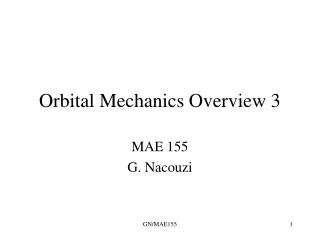DownloadDownload PresentationOrbital Mechanics Overview 3

# Orbital Mechanics Overview 3

Download Presentation## Orbital Mechanics Overview 3

- - - - - - - - - - - - - - - - - - - - - - - - - - - E N D - - - - - - - - - - - - - - - - - - - - - - - - - - -
##### Presentation Transcript

1. Orbital Mechanics Overview 3 MAE 155 G. Nacouzi GN/MAE155

2. Orbital Mechanics Overview 2 • Interplanetary Travel Overview • Coordinate system • Simplifications • Patched Conic Approximation • Simplified Example • General Approach • Gravity Assist: Brief Overview • Project Workshop GN/MAE155

3. Interplanetary Travel: Coordinate System • Use Heliocentric coordinate system,i.e., Sun centered • Use plane of earth’s orbit around sun as fundamental plane, called ecliptic plane • Principal direction, I, is in vernal equinox • Define 1 Astronomical Unit (AU) = semimajor axis of earth orbit (a) = 149.6 E6 km (Pluto, a ~ 39.6 AU) Heliocentric-ecliptic coordinate system for interplanetary transfer: Origin- center of Sun; fundamental plane- ecliptic plane, principal direction - vernal equinox GN/MAE155

4. Principal Forces in Interplanetary Travel • In addition to forces previously discussed, we now need to account for Sun & target planet gravity • Simplify to account only for gravity forces, ignore solar pressure & other perturbances in mission planning => forces considered - Gravity effects of Sun, Earth, Target Planet on S/C GN/MAE155

5. Principal Forces in Interplanetary Travel • A very useful approach in solving the interplanetary trajectory problem is to consider the influence on the S/C of one central body at a time => Familiar 2 body problem • Consider departure from earth: • 1) Earth influence on vehicle during departure • 2) Sun influence=> Sun centered transfer orbit • 3) Target planet gravity influence, arrival orbit GN/MAE155

6. Interplanetary Trajectory Model: Patched Conic • Divide interplanetary trajectory into 3 different regions => basis of the patched-conic approximation • Region 1: Sun centered transfer (Sun gravity dominates) - solved first • Region 2: Earth departure (Earth gravity dominates) - solved second (direct or from parking orbit) • Region 3: Arrival at target planet (planet gravity dominates) - solved third GN/MAE155

7. Interplanetary Trajectory Model: Patched Conic Sphere of Influence • Two body assumption requires calculating the gravitational sphere of influence, Rsoi, of each planet involvedRsoi (radius) = a(planet) x (Mplanet/Msun)^0.4 Earth Rsoi = 1E6 km gravity Rsoi GN/MAE155

8. Interplanetary Trajectory Model: Patched Conic • Sun centered transfer solved first since solution provides information to solve other 2 regions • Consider simplified example of Earth to Venus • Assume circular, coplanar orbits (constant velocity and no plane change needed) • Hohmann transfer used: • Apoapsis of transfer ellipse = radius of Earth Orbit • Periapsis of transfer ellipse = radius of Venus Orbit GN/MAE155

9. Simple Example • Required velocities calculated using fundamental orbital equations discussed earlier • ra= 149.6 km; Va = 27.3 km/s • rp= 108.2 km; Vp = 37.7 km/s • Time of transfer ~146 days Earth @ Launch Venus @ arrival GN/MAE155

10. Simple Example • Example calculations: Hyperbolic Excess Velocity, VHE ,S/C velocity wrt Earth VHE = VS/S - VE/S where, VS/S ~ vel of S/C wrt Sun, VE/S ~ Vel Earth wrt Sun VHE = 27.29 - 29.77 = -2.48 km/s Similarly, @ target planet, hyperbolic excess vel, VHP needs to be accounted for VHP = VS/S - VP/S = 37.7 - 35 = 2.7 km/s; VP/S ~ Vplanet wrt Sun Note: C3 = VHE2, Capability measure of LV GN/MAE155

11. Patched Conic Procedure • 1) Select a launch date based on launch opportunity analysis • 2) Design transfer ellipse from earth to tgt planet • 3) Design departure trajectory (hyperbolic) • 4) Design approach trajectory (hyperbolic) Reference: C. Brown, ‘Elements of SC Design’ GN/MAE155

12. Patched Conic Procedure Mars • 1) Launch opportunityTo minimize required launch energy, Earth is placed (@ launch) directly opposed to tgt planet @ arrival • Calc. TOF, ~ 1/2 period of transfer orbit • Calculate lead angle = Earth angular Vel (e)x TOF • Phase angle, r = 2 pi - lead angle Wait time = r- current/(target - e) r Earth Synodic period~ period between launch opport., S = 2pi/(e - target) S = 2yrs for Mars GN/MAE155

13. Patched Conic Procedure • 2) Develop transfer ellipse from Earth to Target Planet (heliocentric) accounting for plane change as necessary • Note that the transfer ellipse is on a plane that intersects the Sun & Earth at launch, & the target planet at arrival. • Plane change usually made at • departure to combine with injection • and use LV energy instead of S/C GN/MAE155

14. Patched Conic Procedure • 3) Design Departure trajectory to escape Earth SOI, the departure must be hyperbolicwhere, Rpark~ parking orbit, VHE~hyperbolic excess velocity • 4) Design approach trajectory to target planetwhere Vpark is the orbital velocity in the parking orbit and V is the SC velocity at arrival. Vretro is the delta V to get into orbit GN/MAE155

15. Patched Conic Procedure GN/MAE155

16. Gravity Assist Description • Use of planet gravity field to rotate S/C velocity vector and change the magnitude of the velocity wrt Sun. No SC energy is expended Reference: Elements of SC Design, Brown GN/MAE155

17. Gravity Assist Description • The relative velocity of the SC can be increased or decreased depending on the approach trajectory GN/MAE155

18. Examples and Discussion GN/MAE155